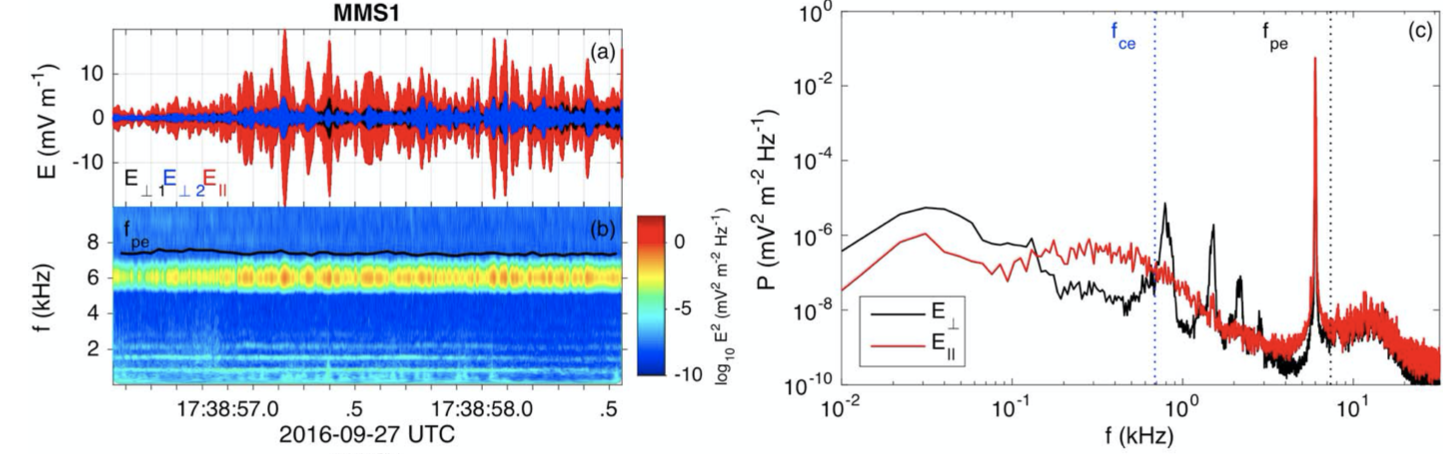# Waves in Plasmas (graduate course)Time: Spring, 2023.

Credit points: 8 ECTS

Prerequisites: Basic course on plasma physics and electromagnetism

Responsible department: Department of Physics and Astronomy, Uppsala University

Teacher: Daniel Graham

First half: Up to kinetic theory.
Second half: Kinetic theory, inhomogeneous waves, nonlinear processes

Reference Book: Plasma Waves, 2nd Edition, D. G. Swanson.
Coursework requires Matlab and software package irfu-matlab (https://github.com/irfu/irfu-matlab).

Learning outcomes:

• Define and explain the concepts of phase velocity and group velocity. Define resonances and cut-offs and explain there physical significance.
• Derive the wave equation for plasmas. Solve the wave equation in fluid plasma and unmagnetised kinetic approximations to calculate the dispersion relations of the supported waves.
• Describe the properties of the different modes described by cold plasma theory. For example, electric and magnetic fields, polarisation properties, and phase and group velocities. Explain how the mode properties change for weakly and strongly magnetised plasmas.
• Be able to analyse waves in spacecraft data. Identify waves in data based on their properties and comparison with theory. Discuss the possible causes of the waves and the possible effects of the waves on the plasma.
• Use numerical dispersion solvers (such as WHAMP) to investigate the growth and damping of waves. Investigate kinetic plasma instabilities numerically, such as the temperature anisotropy instability and streaming instabilities.
Exercises:
Wave Observations project, numerical modelling project, written exam.

## Course Outline

Topic Chapter Concepts
Introduction, Vlasov equations, wave equations, relevant tensors. Swanson 1, most textbooks.
Group velocity and energy transport. Swanson 2.3 Phase/Group velocity.
Cold Plasma waves. Swanson 2.1
Problem 2.1.1
Deriving a conductivity and dialectric tensor. Solve wave equation. Wave dispersion relation.
R, L, O, and X modes. Swanson 2.2
Problem 2.2.2
Problem: What happens if B -> 0?
Wave characteristics: dispersion relation, polarization, cutoff, resonances.
Sub-fci waves: Alfven waves. Swanson 2.5.1 Sheer and compressional Alfven waves.
Whistler waves. Swanson 2.5.2
Problem 2.5.1
Guiding of whistler waves
Electrostatic waves and lower hybrid waves. Swanson 2.8.1—2.8.3
Resonances and particle motion. Swanson 2.9
Fluid plasma waves/MHD waves. Swanson 3.1.1, 3.2 Fluid equation, particle moments, fluid closures, MHD theory. Thermal effects on dispersion relations.
Low-frequency waves in a thermal plasma. Swanson 3.3.1-3.3.3 Ion-acoustic wave. Single fluid approximations
Thermal effects on high-frequency waves. Swanson 3.3.4-3.3.6 Langmuir waves, upper hybrid waves, warm plasma dispersion equation.
Streaming instabilities. Swanson 3.5
XES1 exercise.
Beam-plasma interaction, two-stream instabilities, such as electron-electron and electron-ion.
Kinetic theory of plasma waves: unmagnetized plasmas. Swanson 4.1-4.2
Exercise.
Kinetic wave equation. Langmuir, transverse, and ion-acoustic waves.
Landau solution and Landau damping. Swanson 4.2
Stubbe & Sukhorukov, POP, 1999.
Chen, Ch. 7.
Physical interpretation of Landau damping.
Multi-component plasmas and streaming instabilities Gary, 1993, Ch 3.
Numerical codes
Electron-acoustic waves, beam instability, Buneman instability, ion-ion-acoustic waves.
Evolution of particle distributions Swanson 7.2
XES1 code
Quasilinear diffusion and marginal stability
Kinetic theory of plasma waves: magnetized plasmas. Swanson 4.3
Andre, J. Plasma Phys., 1985
Hot plasma dispersion equation. Electron and ion Bernstein waves.
Temperature anisotropy instabilities: Whistler and Alfven waves Swanson 4.5–4.6
Treumann & Baumjohann 5.2
WHAMP exercise
Wave growth and damping. Cyclotron resonance.
Inertial and kinetic Alfven waves. Stasiewicz et al., Space Sci. Rev., 2000. - Sections 1 and 2.
Inhomogeneous plasma waves. Swanson 6.6
Treumann & Baumjohann 3.1 and 3.3.
Drift waves, e.g., lower-hybrid drift waves. Rayleigh-Taylor and Kelvin-Helmholtz instabilities.
Nonlinear waves, turbulence. Swanson 8.1, 8.3, 8.4 intro. Particle trapping, three-wave processes, etc.
Electromagnetic wave generation. Slides Three-wave processes, linear mode conversion,…